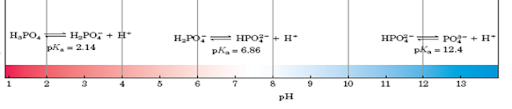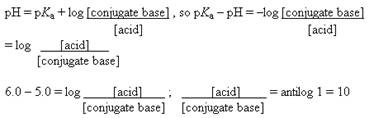# Biology Exams 4 U

Biology Exam Preparation Portal. Preparing with U 4 ur exams...

### pH Practice Problems with Answers

1. Phosphoric acid (H3PO4) has three dissociable protons, with the pKa’s shown below. Which form of phosphoric acid predominates in a solution at pH 4?
Acid    pKa
H3PO4 2.14
H2PO4 6.86
HPO42– 12.4Ans: At pH 4, the first dissociable proton (pKa = 2.14) has been titrated completely, and the second (pKa = 6.86) has just started to be titrated. The dominant form at pH 4 is therefore H2PO4, the form with one dissociated proton.

2. What is the pH of a solution containing 0.2 M acetic acid (pKa = 4.7) and 0.1 M sodium acetate?
Ans:
pH = pKa + log (conjugate base/ acid)
pH = 4.7 + log (0.1/0.2)
= 4.7 – 0.3
pH = 4.4

3. For a weak acid with a pKa of 6.0, show how you would calculate the ratio of acid to salt at pH 5.
Ans:4. Suppose you have just added 100 mL of a solution containing 0.5 mol of acetic acid per liter to 400 mL of 0.5 M NaOH. What is the final pH?
(The pKa of acetic acid is 4.7.)

Ans: Addition of 200 mmol of NaOH (400 mL ´X 0.5 M) to 50 mmol of acetic acid (100 mL X 0.5 mM) completely titrates the acid so that it can no longer act as a buffer and leaves 150 mmol of NaOH dissolved in 500 mL, an [OH] of 0.3 M.
Given [OH], [H+] can be calculated from the water constant:
[H+][OH] = 10–14
[H+] = 10–14 M2 / 0.3 M
pH is, by definition, log (1/[H+])
pH = log (0.3 M /10–14 M2) = 12.48.

5. A weak acid HA, has a pKa of 5.0. If 1.0 mol of this acid and 0.1 mol of NaOH were dissolved in one liter of water, what would the final pH be?
Ans: Combining 1 mol of weak acid with 0.1 mol of NaOH yields 0.9 mol of weak acid and 0.1 mol of salt.
pH = 5.0 + log (0.1/0.9)
pH = 4.05
Please Don't Forget to Share... Thank You... Visit Again...

1.very nice and useful site..please include some notes providing all basic concepts

2.Very nice explanation

We love to hear from you! Leave us a comment.

© Biology Exams 4 U, AllRightsReserved.***Best viewed in Google Chrome and Mozilla firefox***

Maintained by BiologyExams4U.com